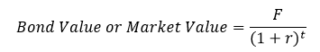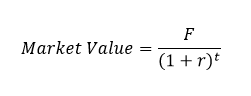Disclaimer: Originally published in March 2017. It is being republished since it still remains an interesting topic till today.

This series will introduce some of the basic (read: very simple) components that make up our financial system and its financial assets. I am not doing this to ridicule our awesome readers but because this series is targeted at people with 0 knowledge of finance whatsoever.

The aim is here to understand the economic environment, financial markets, and the financial instruments that currently exist. We’ll be looking at fixed income securities such as bonds, starting with zero coupon bonds, corporate bonds, treasury securities, etc. We will not be discussing taxation here but you can find some information aboutfrom our previous post.

So next time you talk to your condescending finance major friend, you’ll be able to call them out on their BS or pretend to understand what they are talking about at least.

### What exactly is a Bond?

A bond is a fixed income instrument. It is an IOU, i.e., a note that promises to make certain specified cash flows.

Governments, the Indian Treasury, the US Treasury, corporations, etc. issue bonds to borrow money from investors.

## So it is a piece of paper that gives me money in the future?

Pretty much.

There are two types of cash flows for bonds. There is the Principal or the face value (F) which is the amount of money returned to the investor at maturity.

This face value is also called the Par value and is typically Rs 10, 000 in India and \$1000 in US.  Then there are the coupons, the periodic payments that a bond may make. Coupons are specified by the coupon rate which is expressed as a percentage of the face value.

## Wait, what now?

Face Value =  \$\$\$ you get when the bond matures or completes
Coupons = Periodic \$\$\$ you get, usually twice a year (semi-annually)

## Yeah idk what that means.

Let’s do some math now, shall we?

Face Value, F = \$1000

Coupon Rate = 5%

Payments = \$1000 * 5% = \$50

So you’ll get \$50 annually or \$25 semi-annually which is usually the case.

Notice that the 5% coupon rate doesn’t really tell us anything about the rate of return (benefit) on the bond. So it is meaningless by itself. Coupon rates just tell us what the expected cash flows for a bond are.

## So how do I value bonds?

Now we’re talking. Let’s look at the very basic, super simple Zero-Coupon Bonds.

Zero coupon bonds are probably the simplest financial assets or bonds to value since they only have one cash flow. As the name implies, zero coupon bonds do not have any coupon payments attached with them.  The only cash flow for zero-coupon bonds is the face value at maturity.

## So what’s the value of a zero coupon bond?

The value of a zero coupon bond is simply the discounted value of its face value today. It is just the present value of its 1 single cash flow, today.

## Uhh more math now?

Quit being a baby. Let’s get to it.

What’s the value (market value = how much should you pay for it today) of:

• 1 Year zero-coupon bond (t=1)
• Face value = \$10,000 (F=10,000)
• Discount rate = 5.5% (r=5.5%, the opportunity cost today)So,

Market Value = 10,000/(1.055)1

Market Value = \$9478.67

This is what the price of this bond is today, at the specified discount rate.

## So what’s my profit?

Typically, you would know the market value and the future value (it’s a zero coupon bond, there is only a single cash flow at maturity), so you can quickly calculate the internal rate of return or more commonly called as the yield-to-maturity (YTM).

We do this by finding the interest rate that makes the present value of the face value equal to the market price or the bond price.

## Wait, math again?

You know it.

• 20 Year zero-coupon bond (t=20)
• Face value = \$1,000,000 (F=1,000,000)
• Market value = \$455,500

So if we buy this bond today and hold it till maturity, our expected annual return can be calculated as:Using excel and solving for r, we find that the interest rate would be ~4.01% assuming annual compounding.## So that’s it?

Yup. That’s all to it..for the most part. This was just a simplified case of valuing a zero coupon bond. We’ll be looking at many more elements that make up our financial system.

To stay tuned for another post of Everything Finance, subscribe to our mailing list right now!